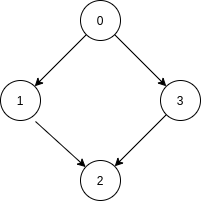# All Paths From Source Lead To Destination

Posted: 27 Mar, 2021
Difficulty: Moderate

## PROBLEM STATEMENT

#### Note :

``````1. The given graph may have self-loops and parallel edges.
``````
##### Example :
``````Consider ‘N’ = 4, ‘EDGES’ = [[0, 1], [0, 3], [1, 2], [3, 2]],  ‘SRC’ = 0 and ‘DEST’ = 2. The given directed graph is shown below.
````````````Here, all the paths that start from node 0 are -:
1. 0->1->2
2. 0->3->2
Both of these paths eventually end at node ‘2’ i.e node ‘DEST’. Thus we should return True.
``````
##### Input format :
``````The first line of input contains an integer ‘T’ denoting the number of test cases. then ‘T’ test cases follow.

The first line of each test case consists of four single space-separated integers  ‘N’, ‘M’, ‘SRC’, ‘DEST’, described in the problem statement.

Then next ‘M’ lines follow in each test case. The ith line consists of two space-separated integers ‘EDGES[i]’ and ‘EDGES[i]’ representing that there is a directed edge from node ‘EDGES[i]’ to ‘EDGES[i]’.
``````
##### Output format :
``````For each test case, print a string “True” if all paths starting from node ‘SRC’ eventually end at node ‘DEST’, Otherwise, print “False”.

Print output of each test case in a separate line.
``````

#### Note :

``````You do not need to print anything, it has already been taken care of. Just implement the given function.
``````
##### Constraints :
``````1 <= T <= 50
2 <= N <= 10^4
0 <= M <= 10^4
0 <= SRC < N
0 <= DEST < N
SRC != DEST
0 <= EDGES[i][j] < N.

Where ‘T’ is the total number of test cases, ‘N’, ‘M’, ‘SRC’, and ‘DEST’ are as described in the problem statement. ‘EDGES[i][j]’ is an integer in list ‘EDGES’.

Time limit: 1 sec
``````Approach 1

All the paths starting from node ‘SRC’ eventually end at node ‘DEST’ if -:

1. Node ‘DEST’ is reachable from ‘SRC’.
2. There is no outgoing edge from node ‘DEST’.
3. No cycle is reachable from ‘SRC’.

We can check these conditions using the Depth First Search algorithm as follows -:

Algorithm

• Create a list of lists ‘ADJ’ of size ‘N’.
• Create an adjacency list of the given directed graph. This can be done by running a loop where ‘i’ ranges from 0 to ‘M’-1 and for each ‘i’ push EDGES[i] in list ADJ[EDGES[i]].
• If ADJ[DEST] is not empty, return false as there should be no outgoing edge from node ‘DEST’.
• Created a boolean array/list VISITED  of size ‘N’, initially fill it with false.
• Created a boolean array/list RECSTK  of size ‘N’ to track recursion stack while DFS, initially fill it with false.
• Run a depth-first search algorithm by calling a recursive function, named  DFS(‘CUR’, ‘DEST’, ‘VISITED’, RECSTK, ‘ADJ’ ). It will return True if all the paths starting from node ‘CUR’ eventually end at node ‘DEST’  In each recursive call of this function, we will do the following steps.
• If ‘CUR’ = ‘DEST’, then return True.
• If ‘ADJ[CUR]’ is empty, then return False, because the path ends at a node other than ‘DEST’.
• Do VISITED[‘CUR’]:= True, and RECSTK[‘CUR’]:= True.
• Iterate over ADJ[‘CUR’] and for each node ‘V’ in it do the following -:
• If RECSTK[‘V’] is True then return False, because the cycle is detected.
• If VISITED[‘V’] is False, then recursively call DFS(‘V’, ‘DEST’, ‘VISITED’ ‘ADJ’ ). Return False, if this recursive call to DFS returns False.
• Do RECSTK[‘CUR’]:= False, and return True.
• Return  DFS(‘SRC’, ‘DEST’, ‘VISITED’, RECSTK, ‘ADJ’ ).